# Basics of Solar Cell Technology

Solar energy has enormous potential and it is highest among all the available sources of energy available whether it is renewable or non renewable sources. It is free, it is renewable and it is clean source of energy.

Assume that you have told to set up a solar power plant in India and you have given sufficient fund and land.  So what you will do??!!

Following are the points which you should think:

1) first you have to find a place in India where maximum solar power is hitting (Solar Ir-radiance ).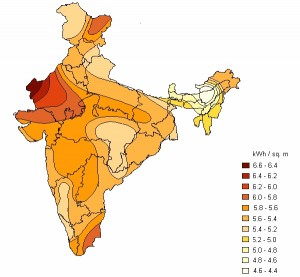2)      Lets say you have decided to put solar power plant in Gujarat. Now in Gujarat you have to see at what location exactly what amount of solar energy is hitting per square area.

Now we will  understand how it is calculated:

Any body which is above 0K  temperature will emit radiation according to planks law which is

given by: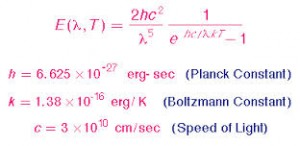Above expression is for a body which is at particular  temperature and emitting radiation of a particular wavelength  but as we know body is  emitting radiations of all wavelengths from 0 to infinity. So we have to integrate that expression E(ƛ)d(ƛ) from o to infinity to find total emissive power emitted by the body.

Similarly consider sun as the source of solar radiations and earth is intercepting those radiations .the amount of solar radiation hitting on some area of earth will depend upon solid angle multiplied total emissive  power of sun.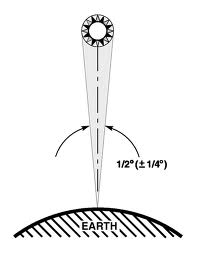So by doing integration of solar irradiance expression (emissive power) multiplied by solid angle of whole earth considering no atmosphere we will get a fix value known as solar flux and that is equal to 1367 W/m2.

Solar flux: The total energy flux (energy per time per area) incident on a unit area perpendicular to a beam outside the Earth’s atmosphere.

Air mass factor(AM): Air mass factor gives you idea about the relative position of sun w.r.t. to earth.

AM = 1/ CosƟ , where Ɵ equal to angle from vertcle line when sun is directly overhead to us.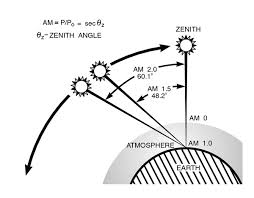For e.g.  AM 1.5 = 1/CosƟ  => Ɵ=48.2*

AM0 means no atmosphere.

Now you have to calculate the solar flux which is hitting on your area in Gujarat. What you will do you will first of all find latitude of that area.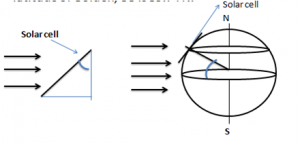After finding latitude we have to multiply that solar power density which is coming parallel to plane of equator with cosine of latitude because we want those photons which are hitting perpendicular to our solar cell.

3) So we have decided the location of power plant by calculating the exact solar flux hitting that area.

4) Now what next?? Before installing the solar panels we have to understand how solar cell works:

Working

• The main part of solar cell is p-n junction. When p type and n type material are joined there is formation of space charge region/depletion region and It stays localized at the P-N junction and an electric field has been created.
• If the solar cell is put in the sun, photons will strike the surface of the Silicon and pass their energy on to electrons. A typical photon can eject one electron from its nucleus creating a free electron and a vacancy. These free electrons will feel the effect of the electric field. They are pushed towards the junction on the N-side and away from the junction on the P-side. Likewise, the vacancy, which has a net positive charge, will be pulled towards the junction on P-side and pushed away from it on the N-side.
• So there is current flowing from p type to n type material. This current is known as photo induced current and denoted by IL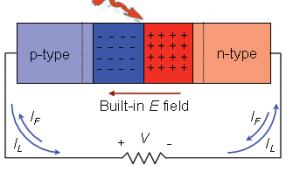• We can see from the graph that there is point where we are getting maximum power. so our aim is to find that point. This can be easily calculated by simple differentiation technique.
• If still not clear , watch this video: https://www.youtube.com/watch?v=1gta2ICarDw

Efficiency

• Efficiency is most important thing to any type energy system. We have reached maximum efficiency of  44% in case of solar cell.(search and find what are the efficiency of other sources of energy)
• How to find efficiency of our solar cell???…

To find efficiency we have to first follow above procedure and calculate Jmax and Vmax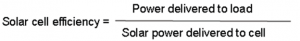Above numerator term is Jmax* Vmax

Denominator is solar power denoted by Ps   at some AM value.

For e.g. Ps value at AM= 1.5 means

1367W/m2 *cos(41.8) =1000 W/m2

5) After learning basics of solar cell, let’s build solar cell. Before we discuss the components of real solar cell,( just think what can be most imp building blocks of solar cell !!!  )

Following are the main components of solar cell:

• P-n junction : Every p-n system is characterized by its unique band bap( ΔEg) or    forbidden energy gap.
• Anti Reflecting Coating:  If ray of light incident on any surface ,some part is reflected and some part is transmitted.  we have to have maximize the transmittance .so we are applying the layer of such a material whose refractive index is the geometric mean of mediums which are above and below of anti reflective coating.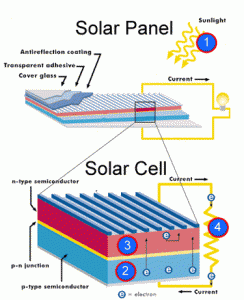• Glass: glass is used for two purposes first to concentrate the beam of light secondly to have self cleaning property so that any dust particle or any organic impurity can be washed off easily.

So to have self cleaning property of glass, two methods are used:

1. We can coat a hydrophobic layer on glass which will make the contact angle for water – glass system to very large and consequently a spherical drop of water will from, which rolls down due to gravity taking away dust .
2. We can coat a super hydrophilic coating on glass e.g. coating of TiO2. The glass cleans itself in two stages. The “photo catalytic” stage of the process breaks down the organic dirt on the glass using ultraviolet light and makes the glass super hydrophilic (normally glass is hydrophilic. During the following “super hydrophilic” stage, rain washes away the dirt, leaving almost no streaks, because water spreads evenly on super hydrophilic surfaces
• Conducting wires: these are used to connect small -small solar cells into series and parallel.

Watch the below link to know how solar cell is manufactured. This video is of company- ‘’Sun Tech Power”.

6) Now you have installed solar cells and production is started. Now you have to know what maintenance is required and what are the factors which affects the performance of my cell.

Following are the factors which affects the efficiency of solar cell:

• Loss of photons which are below the band gap:

This loss is highest among all losses. We know that light which is hitting contains photons of various energies but out of 100% photons only 30% are capable of generating current because a p-n junction is fixed for photon which have energy equal to band width rest are of no use.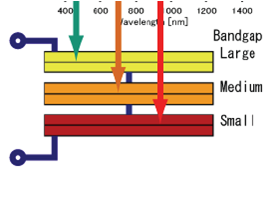There are multiple p-n junction kept one below the another and of variable band gaps.

• Loss of energy from relaxation of carriers to band edges:

To understand this lets go to atomic level of p type material. This material when irradiated with some light electron will make jump from valence band to conduction band. Now this electron from the conduction band of p type goes to conduction band of n type via space charge region and during this journey electron losses its potential energy and relax at the band edges which results in dissipation of heat   as shown in fig: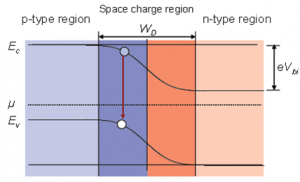• Resistance losses (shunt and series resistances) which will decrease Voc and photo current:

This is the second largest loss after loss of photons. Generally two types of resistances are there series and shunt .These resistances arises because of:

Series: 1. Resistence of connecting wires.

2. Contact resistances.

Shunt (parallel) : These are mainly due to impurities phases lying from  p type to  n type material .

•  Junction recombination: electrons which are generated by light, some of them will lost while going through depletion region.
• Optical losses :
• Dirt accumulation on the glass :
• Effect of temperature:  There is optimum range of temperature in which solar cell works efficiently ,Increasing temperature will decrease the cell efficiency.
• Carrier recombination at defects: This effect is due to grain boundaries resulting in decrement of light current.

7) At last these are materials used in solar cell technology with their efficiencies: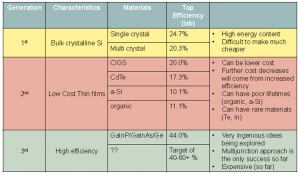In India we have largest solar park of Asia at Charanka village, Patan district, Gujarat. There is video on it : https://www.youtube.com/watch?v=kIhHy8X0kCg

Refrences:

Stanford university free online course: “solar cell, fuel cell and batteries”

CEV - Handout